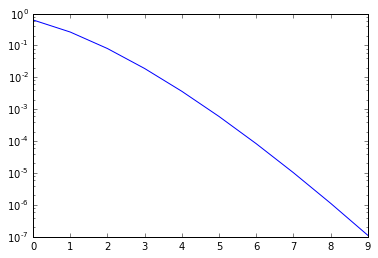# Floating Point Arithmetic and the Series for the Exponential Function¶

In :
import numpy as np
import matplotlib.pyplot as pt


What this demo does is sum the series $$\exp(x) \approx \sum_{i=0}^n \frac{x^i}{i!},$$ for varying $n$, and varying $x$. It then prints the partial sum, the true value, and the final term of the series.

In :
a = 0.0
x = 1e0 # flip sign
true_f = np.exp(x)
e = []

for i in range(0, 10): # crank up
d = np.prod(
np.arange(1, i+1).astype(np.float))

# series for exp
a += x**i / d

print(a, np.exp(x), x**i / d)

e.append(abs(true_f-a)/true_f)

1.0 2.71828182846 1.0
2.0 2.71828182846 1.0
2.5 2.71828182846 0.5
2.66666666667 2.71828182846 0.166666666667
2.70833333333 2.71828182846 0.0416666666667
2.71666666667 2.71828182846 0.00833333333333
2.71805555556 2.71828182846 0.00138888888889
2.71825396825 2.71828182846 0.000198412698413
2.71827876984 2.71828182846 2.48015873016e-05
2.71828152557 2.71828182846 2.7557319224e-06

In :
pt.semilogy(e)

Out:
[<matplotlib.lines.Line2D at 0x7f0adc77f4a8>]